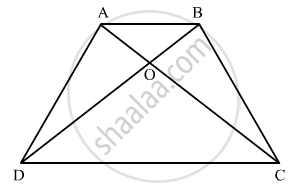Advertisement Remove all ads

# Abcd is a Trapezium Having Ab || Dc. Prove that O, the Point of Intersection of Diagonals, Divides the Two Diagonals in the Same Ratio. Also Prove that a R ( δ O C D ) a R ( δ O a B ) = 1 9 If Ab - Mathematics

Sum

ABCD is a trapezium having AB || DC. Prove that O, the point of intersection of diagonals, divides the two diagonals in the same ratio. Also prove that

$\frac{ar\left( ∆ OCD \right)}{ar\left( ∆ OAB \right)} = \frac{1}{9}$ if AB = 3 CD.
Advertisement Remove all ads

#### Solution

We are given ABCD is a trapezium with AB||DCConsider the triangles AOB and COD in which

∠ AOB = ∠COD

∠ ABO = ∠ODC  (ALTERNATIVE ANGLE)

∠ BAO = ∠DCA  (ALTERNATIVE ANGLE)

Therefore,  ∆ODC ∼ ∆ OBA

⇒ (AO)/(OC)=(BO)/(DO)=(AB)/(CD)

⇒ (AO)/(OC)=(BO)/(DO)

Hence we have proved that O, the point of intersection of diagonals, divides the two diagonals in the same ratio.

We are given AB = 3CD and we have to prove that (ar∆ OCD)/(ar∆ OAB)=1/9

We already have proved that AOB and COD are similar triangles

So

(ar∆ OCD)/(ar∆ OAB)= (CD^2)/(AB^2)

(ar∆ OCD)/(ar∆ OAB)= (CD^2)/(3CD^2)

(ar∆ OCD)/(ar∆ OAB)= 1/9

Hence, Prove that  (ar∆ OCD)/(ar∆ OAB)= 1/9

Concept: Triangles Examples and Solutions
Is there an error in this question or solution?
Advertisement Remove all ads

#### APPEARS IN

RD Sharma Class 10 Maths
Chapter 7 Triangles
Q 16 | Page 126
Advertisement Remove all ads

#### Video TutorialsVIEW ALL 

Advertisement Remove all ads
Share
Notifications

View all notifications

Forgot password?
Course